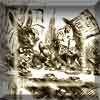#### You may also like### A Problem of Time

Consider a watch face which has identical hands and identical marks for the hours. It is opposite to a mirror. When is the time as read direct and in the mirror exactly the same between 6 and 7?### Fit for Photocopying

Explore the relationships between different paper sizes.### Back in Time

How many times a day does a 24 hour digital clock look the same when reflected in a horizontal line?

# Similar Perimeter

##### Age 14 to 16 ShortChallenge Level

To get a side length of 12,

• the side of length 3 could be enlarged by a scale factor of 4
or
• the side of length 4 could be enlarged by a scale factor of 3
or
• the side of length 6 could be enlarged by a scale factor of 2

The perimeter of triangle A is 3 + 4 + 6 = 13, so the possible perimeters of triangle B are:

12 + 16 + 24 = 52 (following an enlargement by a scale factor of 4)
or
9 + 12 + 18 = 39 (following an enlargement by a scale factor of 3)
or
and 6 + 8 + 12 = 26 (following an enlargement by a scale factor of 2)

You can find more short problems, arranged by curriculum topic, in our short problems collection.